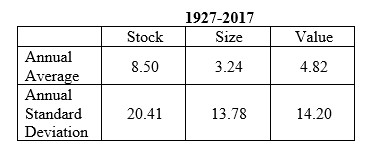# Swedroe: Be Prepared For Losses

October 03, 2018

The stock premium—the annual average return of stocks minus the annual average return of one-month Treasury bills—has been high, attracting investors to the stock market. For the period 1927–2017, it averaged 8.5%. There have also been size (return of small stocks minus return of large stocks) and value (return of value stocks minus return of growth stocks) premiums of 3.24% and 4.82%, respectively.

However, the excess returns are generally referred to as risk premiums—they aren’t free lunches. We see evidence of that in the volatility of the premiums. The stock, size and value premiums have come with annual standard deviations of 20.41% (2.4 times the stock premium), 13.78% (4.3 times the size premium) and 14.20% (2.9 times the value premium), respectively.Let’s take a closer look at some of the data that illustrate the riskiness of the premiums. For the period:

• The stock premium was negative in 27 of the 91 years (30% of the years). There were 17 years (19% of the years) when the premium was worse than -10%, 12 years (13% of the years) when it was worse than -15% and seven years (8% of the years) when it was worse than -20%. As another indicator of the volatility of the stock premium, we see that the gap between the best and worst years was 102.2%, more than 12 times the size of the premium itself. The worst year was 1931, when the premium was -45.11%. Given a premium of 8.5% and a standard deviation of 20.41%, this was more than a 2.5 standard deviation event. The best year was 1933, when the premium was 57.05%, also more than two standard deviations from the mean. In fact, we had six such two-standard-deviation events. We would have had just four if the returns had been normally distributed.
• The size premium was negative in 42 of the years (46% of the years). There were 10 years (11% of the years) when it was worse than -10%, three years (3% of the years) when it was worse than -15%, and three years (3% of the years) when it was worse than -20%. The worst year was 1929, when the premium was -30.8%, a 2.5-standard-deviation event. The best year was 1967, when the premium was 50.69%, more than a three-standard-deviation event. The gap between the best and worst years was 81.49%, more than 25 times the size of the premium itself. In five of the years, returns were more than two standard deviations from the mean.
• The value premium was negative in 35 of the years (38% of the years). There were 11 years (12% of the years) when it was worse than -10%, four years (4% of the years) when it was worse than -15%, and three years (3% of the years) when it was worse than -20%. The worst year was 1999, when the premium was -31.7%, more than a 2.5-standard-deviation event. The best year was 2000, when the premium was 39.69%, a 2.5-standard-deviation event. The gap between the two was 71.39%, 14.8 times the size of the premium itself. In six of the years, returns were more than two standard deviations from the mean. Even if we extend our time frame to five years, we see that there is still risk in the premiums. Using non-overlapping data, we have 18 five-year periods.
• In four periods (22% of the periods), the stock premium was negative. The worst period was 1927–31, when the stock premium was -46.76%.
• In nine periods (50% of the periods), the size premium was negative. The worst period was also 1927–31, when it was -30.92%.
• In five periods (28% of the periods), the value premium was negative. The worst period was 2007–11, when it was -32.27%. If we extend our time frame to 10 years, we have nine non-overlapping periods. There were no periods when the stock premium was negative, one period when the value premium was negative (2007–16, when it was -17.59%) and two periods when the size premium was negative (1947–56, when it was -29.95% and 1987–96, when it was -19.21%).